Next: Bayesian inference for simple Up: Rules of probability Previous: Probability of complete classes

## Probability rules for uncertain variables

In analyzing the data from physics experiments, we need to deal with measurement that are discrete or continuous in nature. Our aim is to make inferences about the models that we believe appropriately describe the physical situation, and/or, within a given model, to determine the values of relevant physics quantities. Thus, we need the probability rules that apply to uncertain variables, whether they are discrete or continuous. The rules for complete classes described in the preceding section clearly apply directly to discrete variables. With only slight changes, the same rules also apply to continuous variables because they may be thought of as a limit of discrete variables, as interval between possible discrete values goes to zero.

For a discrete variable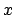, the expression, which is called a probability function, has the interpretation in terms of the probability of the proposition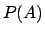, whereis true when the value of the variable is equal to. In the case of continuous variables, we use the same notation, but with the meaning of a probability density function (pdf). So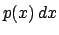, in terms of a proposition, is the probability, whereis true when the value of the variable lies in the range ofto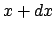. In general, the meaning is clear from the context; otherwise it should be stated. Probabilities involving more than one variable, like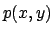, have the meaning of the probability of a logical product; they are usually called joint probabilities.

Table 1 summarizes useful formulae for discrete and continuous variables. The interpretation and use of these relations in Bayesian inference will be illustrated in the following sections.

 discrete variables continuous variables probability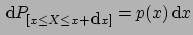normalizationexpectation of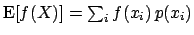expected value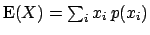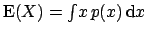moment of order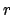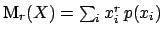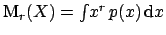variance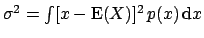product rule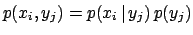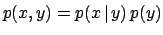independence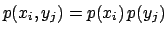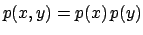marginalizationdecomposition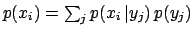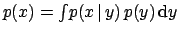Bayes' theorem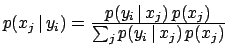likelihood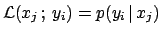A functionsuch that, or, is called improper. Improper functions are often used to describe relative beliefs about the possible values of a variable.Next: Bayesian inference for simple Up: Rules of probability Previous: Probability of complete classes
Giulio D'Agostini 2003-05-13